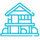# Advanced hydro - nutrient calculations

baci
8 years ago

emmaF 5/6/04 at 17:25

I am looking to find out what how some fertilizers are made. The derived from list is written, i.e. derived from EDTA chelates of Manganese, Potassium Nitrate etc., and the analysis of the % of Nitrogen is written and divided into what types, but of course the chemical compounds and proportions used are secret. I have looked for information on this online but only can find results from some states metals testing of fertilizers. The fertilizer companies will not reveal this information. Is there a way I can get a near accurate idea? I have trouble enough typing, so I dont think I can figure this one out on my own. Any suggestions will be great! Thanks

emmaF 5/23/04 at 20:09

I am looking to find out specifically about a few of the most popular liquid hydroponic nutrients on the market. How much of each ingredients do they use to get the final product. Ingredients are listed but the amounts/ method are almost always a trade secret. Thank you for all the leads, I will check them out now.

emmaF 5/26/04 @ 1:57

I would like to make a recipe using the following:

Amonium Nitrate
Magnesium Nitrate
Potassium Nitrate
Magnesium Phosphate
Potassium Phosphate
Potassium Carbonate
Potassium Sulfate
Magnesium Carbonate
Magnesium Sulfate

Ending with a liquid fertilizer with an analysis of 2-1-6 (with0.5Mg)
Using these things what might be the correct proportion in % or grams --roughly o.k. also--would be needed.

Response: DHershey 7/24/04 @ 21:15

Fertilizer formulas are not secret. All the information needed is on the label. You just have to know how to do the calculations, which are rather challenging.

The ratio you have is very strange, N is far too low relative to P and K. Despite that, here's one of several ways you could make one liter of your 2-1-6 solution.

First you have to convert the 2-1-6, which is an archaic N-P2O5-K2O, to N-P-K.

P2O5 x 0.43 = %P
K2O x 0.83 = %K

After conversion, you have 2N-0.43P-4.98K

I'm assuming you want to use all nitrate-N. You need to know the elemental percentages for each of your fertilizer salts. They should be on the fertilizer container. Remember to convert P2O5 and K2O to P and K.

1. Start with the 0.5 Mg and use magnesium nitrate - Mg(NO3)2 6H2O - which has a molecular weight of 256.41. The molecular weight of Mg is 24.3 so 24.3/256.41 x 100 = 9.5 % Mg

Multiply the percentage required by 10,000 to get mg/liter. The 0.5 Mg is roughly equivalent to 5,000 mg/liter or 5 g/liter.

To determine how much magnesium nitrate is required to give 5 g Mg, divide 5 by the %Mg in magnesium nitrate written as a decimal. So 5/0.095 =

52.6 g magnesium nitrate per liter 2. Determine how much nitrogen is supplied by magnesium nitrate. The percent N in magnesium sulfate is 28/256.41 x 100 = 10.9% so the 52.6 g magnesium nitrate provides 0.109 x 52.6 = 5.7 g N

3. Use monopotassium phosphate to provide the 0.43P. Monopotassium phosphate (KH2PO4) contains about 22.3%P and 28.2%K

Multiply 0.43 x 10,000 = 4,300 mg/liter = 4.3 g/liter
4.3/0.223 =

19.3 g monopotassium phosphate 4. Determine how much K was provided by monopotassium phosphate.
19.3 g x 0.282 = 5.44 g K

5. Use potassium nitrate (KNO3), which is 13% N and 37% K, to provide the rest of the N.

The 2%N represents 20,000 mg/liter N or 20 g/liter.
From step 2, you already have 5.7 g N supplied by magnesium nitrate.

Therefore, you only need 20 - 5.7 = 14.3 g N.
Take 14.3/0.13 =

110 g potassium nitrate 6. Determine how much K was provided by potassium nitrate.
110 x 0.37 = 40.7 g K.

7. For potassium, you need 4.98 x 10,000 = 49,800 mg/liter = 49.8 g/liter

From step 4, you provided 5.44 g K
From step 6, you provided 40.7 g K.

Therefore, you only need 49.8 - 5.44 - 40.7 = 3.66 g K

Potassium sulfate K2SO4 contains about 44%K
3.66/0.44 =

8.3 g potassium sulfate dontsleep 10 7/1/06 @ 13:37

If you are trying to reverse engineer a product consider that in the USA, by definiton, "guaranteed analysis" is the MINIMUM percentage of each plant nutrient claimed. Proprietary surfectants and stabilizers will not be listed. The source and quality of the ingredients will factor as well. Thus your results will vary.